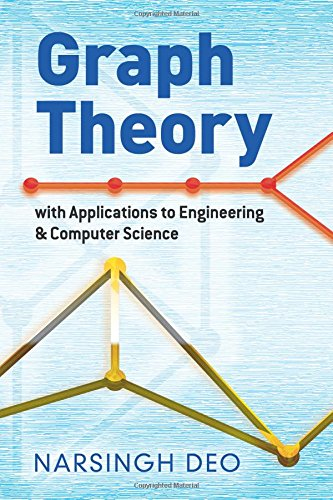# Graph Theory with Applications to Engineering and Computer Science (Dover Books on Mathematics)

Price: points - Details)

This outstanding introductory treatment of graph theory and its applications has had a long life in the instruction of advanced undergraduates and graduate students in all areas that require knowledge of this subject. The first nine chapters constitute an excellent overall introduction, requiring only some knowledge of set theory and matrix algebra. Topics include paths and circuits, trees and fundamental circuits, planar and dual graphs, vector and matrix representation of graphs, and related subjects.
The remaining six chapters are more advanced, covering graph theory algorithms and computer programs, graphs in switching and coding theory, electrical network analysis by graph theory, graph theory in operations research, and more. Instructors may combine these chapters with the preceding material for courses in a variety of fields, including electrical engineering, computer science, operations research, and applied mathematics.Graph Theory with Applications to Engineering and Computer Science (Dover Books on Mathematics)